Math Functions in JavaScript || JavaScript Tutorials# Math Functions in JavaScript

2392

Sometimes there is a need for accomplishing mathematical tasks in various web-projects for which developers use JavaScript Math function objects.

Now, what are Math function objects?

The concepts of objects are similar in other programming languages. If you are not familiar with objects, go through the previous topics of JavaScript tutorial published by Coding Tag or click on the below-given direct link.

https://www.codingtag.com/javascript-objects

Math function objects are the inbuilt functions present in JavaScript which permits the developer to perform mathematical activities such as finding round up functions, square roots, minimum or maximum numbers, etc.

Let's take a real-time example of Math function in JS.

Math.PI;// returns 3.141592

In JavaScript, there is no need for writing the value of PI multiple times as it has already provided the constant i.e. "Math.PI;" This constant returns the value of PI i.e. 3.141599265

I hate mathematics. But I can't deny that mathematical is a prime part of academics. In programming, there are many situations that may come where we have to rely on mathematical tasks.

I was developing my B.tech project. Meanwhile, there was a need to process numerical information for performing some calculations. That situation tend me to search for the shortcuts of mathematical tasks in programming.

At last, I found there are inbuilt mathematical functions in JavaScript. These functions have helped me to perform my difficult task effortlessly.

Benefits of Math Functions:

i) Math objects permit efficient calculations in JavaScript.

ii) The developers will save their time because they do not need to spend their time on accessing properties and methods of Math object to perform a task on numbers.

These methods can be directly accessed through a JavaScript interpreter.

JavaScript Math Objects

There are several math objects which have been found in JavaScript. Some are given below:

i) Math.round()

ii) Math.pow()

iii) Math.sqrt()

iv) Math.random()

v) Math.ceil()

Math.abs()

If there is a need to return the number's absolute value while programming in JavaScript, then Math.abs() function can be implemented. This function receives the numbers like their parameters and then further returns their absolute values as in the following example.

```<!DOCTYPE html>
<html>
<body>

<h2> Math.abs() in JS</h2>

<p>A simple example illustrating Math.abs(x) returning the absolute value in the output:</p>
<p id="demo"></p>

<script>
document.write(Math.abs(54+67));
</script>

</body>
</html>```

Output: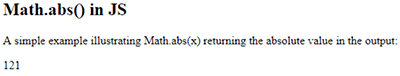The syntax which has been used while writing the above-mentioned program of Math.abs is written below:

`Math.abs(value)`

math.ceil() function

math.ceil() function is applicable in the conditions when there is a need to return the smallest numerical values greater than a given number in the module. In other words, we can say to round up any numeric numbers to their nearest value towards the upward direction, One can use math. ceil function.

Syntax:

`Math.ceil(value)`

Program:

```<!DOCTYPE html>
<html>
<body>

<h2> Math.ceil() in JavaScript </h2>

<p>Math.ceil() roundup a numeric number <strong>towards</strong> its nearest integer value:</p>
<p id="demo"></p>

<script>
document.write(Math.ceil(-5) + "<br>");
document.write(Math.ceil(-5.67));
</script>

</body>
</html>```

The output appeared as given below: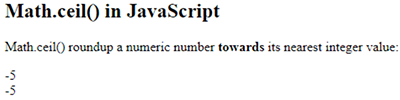Math.round() function

JavaScript Math.round() function returns a rounded value of a certain number to their nearest integers.

Syntax:

`Math.round(variable);`

Program:

```<!DOCTYPE html>
<html>
<body>

<h2> Math.round() in JavaScript </h2>

<p> A simple program demonstrating the rounding of the numbers </p>
<p id="demo"></p>

<script>
var roundingnumber =Math.round(9.1);
document.write("After rounding the number : " + roundingnumber);
</script>

</body>
</html>```

The output being appeared while executing the above program is given below: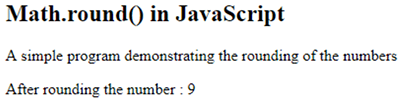Math.pow()

JavaScript Math.pow() function is used to compute the power of a specified expression in a code.

Syntax

`Math.pow(P, Q);`

The above-mentioned syntax consists of a base (P) and exponent (Q) to represent base and exponent values respectively.

Following in the program of JavaScript with pow():

```<!DOCTYPE html>
<html>
<body>

<script>
document.writeln(Math.pow(7,9)+"<br>");
document.writeln(Math.pow(8,2.7));
</script>

</body>
</html>```

Math.sqrt()

In JavaScript, the square root of any number is being calculated through Math.sqrt() function. The calculated value is passed to the function as a parameter.

Syntax:

`Math.sqrt(value)`

Use Case:

```<!DOCTYPE html>
<html>
<body>

<h2> Math.sqrt() in JavaScript</h2>

<p>A simple example of Math Function performing square root:</p>

<p id="demo23"></p>

<script>
document.getElementById("demo23").innerHTML = Math.sqrt(98);
</script>

</body>
</html>```

Output of the above-written program has been appeared as: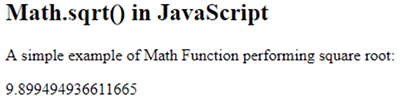Math.random()

If you want to find the random number between the range i.e. 0 or 1 in JavaScript then use the Math.random() through the syntax which is given below:

Math.random()

Let's understand the concept of Math.random() through a simple program

```<!DOCTYPE html>
<html>
<body>

<h2> Math.random() in JavaScript</h2>

<p>A simple program of Math.random() in JavaScript :</p>

<p id="demo"></p>

<script>
var rand1 = Math.random( );
document.write("The random number generated through random function: " + rand1 );
</script>

</body>
</html>```

The output appeared as: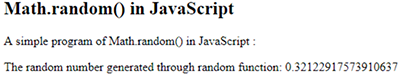Math constants in JavaScript

Math Object in JavaScript permits 8 mathematical constants. Following is the list :

i) Math.LOG2E

ii) Math.E

iii) Math.PI

iv) Math.LN2

v) Math.LOG10E

vi) Math.SQRT2

vii) Math.LN10

viii) Math.SQRT1_2

That's all about Math functions. There are many other Math functions that are available in JavaScript. Through this post, I have discussed major ones.

If you want any other functions to get explained, please comment below . I will surely revert back to you accordingly.

Thank you!!Share:## Get a .COM for just \$6.98

Secure Domain for a Mini Price